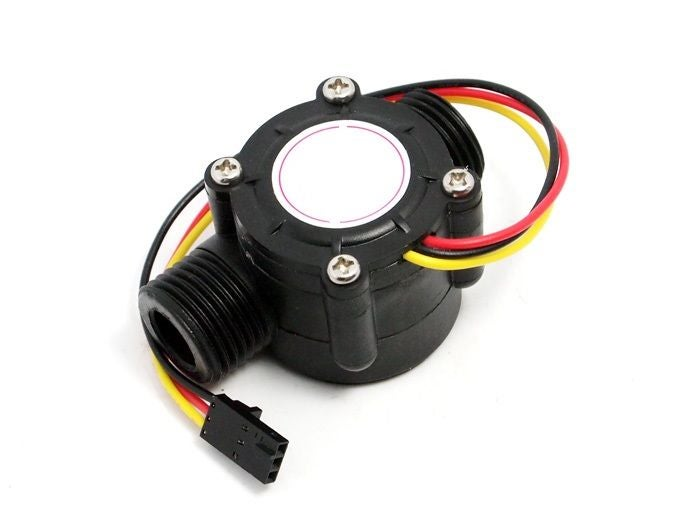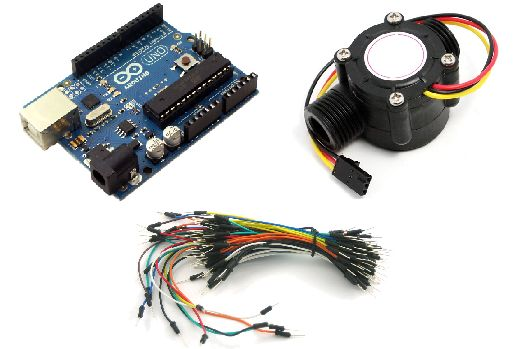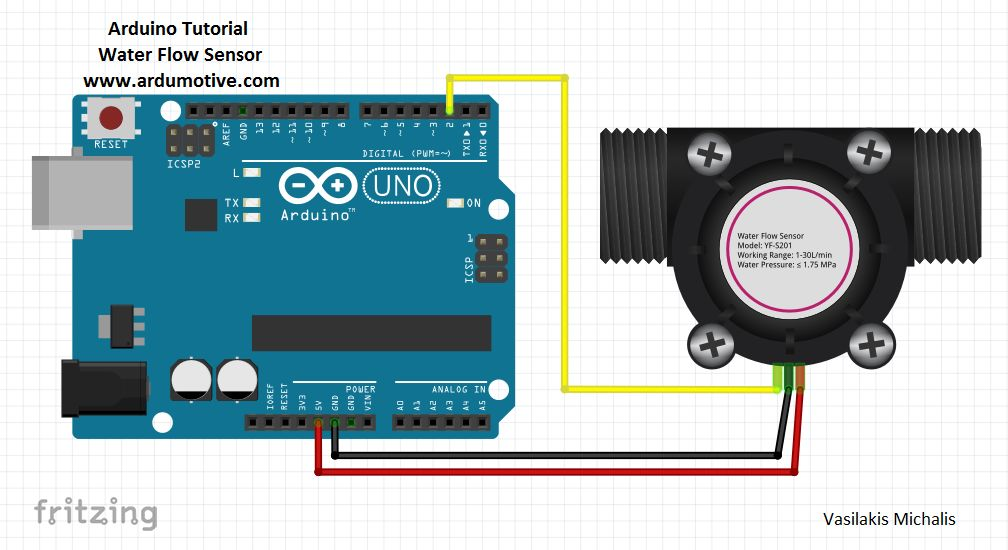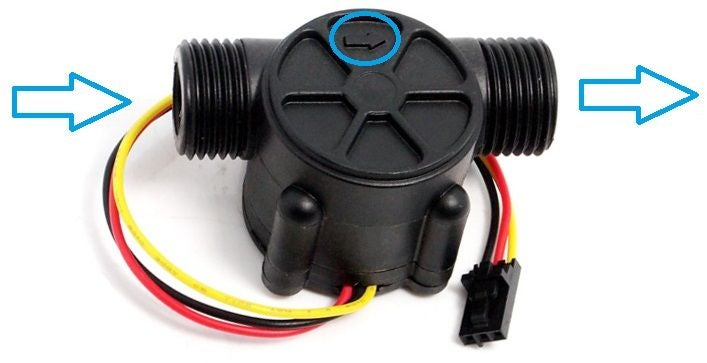# 如何使用水流量传感器?Arduino Uno

3条面包板电缆/*
Liquid flow rate sensor -DIYhacking.com Arvind Sanjeev

Measure the liquid/water flow rate using this code.
Connect Vcc and Gnd of sensor to arduino, and the
signal line to arduino digital pin 2.

*/

byte statusLed    = 13;

byte sensorInterrupt = 0;  // 0 = digital pin 2
byte sensorPin       = 2;

// The hall-effect flow sensor outputs approximately 4.5 pulses per second per
// litre/minute of flow.
float calibrationFactor = 4.5;

volatile byte pulseCount;

float flowRate;
unsigned int flowMilliLitres;
unsigned long totalMilliLitres;

unsigned long oldTime;

void setup()
{

// Initialize a serial connection for reporting values to the host
Serial.begin(9600);

// Set up the status LED line as an output
pinMode(statusLed, OUTPUT);
digitalWrite(statusLed, HIGH);  // We have an active-low LED attached

pinMode(sensorPin, INPUT);
digitalWrite(sensorPin, HIGH);

pulseCount        = 0;
flowRate          = 0.0;
flowMilliLitres   = 0;
totalMilliLitres  = 0;
oldTime           = 0;

// The Hall-effect sensor is connected to pin 2 which uses interrupt 0.
// Configured to trigger on a FALLING state change (transition from HIGH
// state to LOW state)
attachInterrupt(sensorInterrupt, pulseCounter, FALLING);
}

/**
* Main program loop
*/
void loop()
{

if((millis() - oldTime) > 1000)    // Only process counters once per second
{
// Disable the interrupt while calculating flow rate and sending the value to
// the host
detachInterrupt(sensorInterrupt);

// Because this loop may not complete in exactly 1 second intervals we calculate
// the number of milliseconds that have passed since the last execution and use
// that to scale the output. We also apply the calibrationFactor to scale the output
// based on the number of pulses per second per units of measure (litres/minute in
// this case) coming from the sensor.
flowRate = ((1000.0 / (millis() - oldTime)) * pulseCount) / calibrationFactor;

// Note the time this processing pass was executed. Note that because we've
// disabled interrupts the millis() function won't actually be incrementing right
// at this point, but it will still return the value it was set to just before
// interrupts went away.
oldTime = millis();

// Divide the flow rate in litres/minute by 60 to determine how many litres have
// passed through the sensor in this 1 second interval, then multiply by 1000 to
// convert to millilitres.
flowMilliLitres = (flowRate / 60) * 1000;

// Add the millilitres passed in this second to the cumulative total
totalMilliLitres += flowMilliLitres;

unsigned int frac;

// Print the flow rate for this second in litres / minute
Serial.print("Flow rate: ");
Serial.print(int(flowRate));  // Print the integer part of the variable
Serial.print("L/min");
Serial.print("\t");   // Print tab space

// Print the cumulative total of litres flowed since starting
Serial.print("Output Liquid Quantity: ");
Serial.print(totalMilliLitres);
Serial.println("mL");
Serial.print("\t");   // Print tab space
Serial.print(totalMilliLitres/1000);
Serial.print("L");

// Reset the pulse counter so we can start incrementing again
pulseCount = 0;

// Enable the interrupt again now that we've finished sending output
attachInterrupt(sensorInterrupt, pulseCounter, FALLING);
}
}

/*
Insterrupt Service Routine
*/
void pulseCounter()